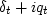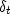Next: Instantaneous envelope Up: HILBERT TRANSFORM Previous: The quadrature filter

## The analytic signal

The so-called analytic signal can be constructed from a real-valued time series ut and itselfphase shifted, i.e., vt can be found using equation (5). The analytic signal is gt, where(11)
In the time domain, the filter [1+ iQ(Z)] is,whereis an impulse function at time t=0. The filtervanishes for negative.Thus it is a real step function in the frequency domain. The values all vanish at negative frequency.

We can guess where the name analytic signal'' came from if we think back to Z-transforms and causal functions. Causal functions are free of poles inside the unit circle, so they are analytic'' there. Their causality is the Fourier dual to the one-sidedness we see here in the frequency domain.

 A function is analytic'' if it is one-sided in the dual (Fourier) domain.Next: Instantaneous envelope Up: HILBERT TRANSFORM Previous: The quadrature filter
Stanford Exploration Project
10/21/1998